×#### Thank you for registering.

One of our academic counsellors will contact you within 1 working day.

Click to Chat

1800-1023-196

+91-120-4616500

CART 0

• 0

MY CART (5)

Use Coupon: CART20 and get 20% off on all online Study Material

ITEM
DETAILS
MRP
DISCOUNT
FINAL PRICE
Total Price: Rs.

There are no items in this cart.
Continue Shopping• Complete JEE Main/Advanced Course and Test Series
• OFFERED PRICE: Rs. 15,900
• View Details

Chapter 11: Time and Work Exercise – 11.1

Question: 1

Rakesh can do a piece of work in 20 days. How much work can he do in 4 days?

Solution:

It is given that:-

Rakesh can do a piece of work in 20 days.

So, Rakesh’s 1 day’s work = 1: 20

Rakesh’s work for 4 days = 4: 20 = 1: 5

Thus, he can do 1/5 th of the work in 4 days.

Question: 2

Rohan can paint 1/3 of a painting in 6 days. How many days will he take to complete the painting?

Solution:

It is given that:

Rohan can paint 1/3 of a painting in 6 days.

So, Time taken by Rohan to complete the painting = 6 × 3 days = 18 days

Question: 3

Anil can do a piece of work in 5 days and Ankur in 4 days. How long will they take to do the same work, if they work together?

Solution:

It is given that:

Time taken by Anil to do the work = 5 days

Time taken by Ankur to do the work = 4 days

So,

Work done by Anil in 1 day = 1: 5

Work done by Ankur in 1 day = 1: 4

So,

Work done by Anil and Ankur in 1 day = 1: 5 + 1: 4 = (4 + 5): 20 = 9: 20

Thus,

Anil and Ankur can do the work in 20: 9 days, i.e., 2(2/9) days.

Question: 4

Mohan takes 9 hours to mow a large lawn. He and Sohan together can mow it in 4 hours. How long will Sohan take to mow the lawn if he works alone?

Solution:

It is given that:

Time taken by Mohan to mow the lawn = 9 hours

Time taken by Mohan and Sohan to mow the lawn = 4 hours.

So,

Work done by Mohan = 1: 9

Work done by Mohan and Sohan = 1: 4

Work done by Sohan = 1: 4 – 1: 9

= (9 – 4): 36

= 5: 36

Thus,

Sohan can do the work in 36: 5 hours, i.e., 7(1/5) hours.

Question: 5

Sita can finish typing a 100 page document in 9 hours, Mita in 6 hours and Rita in 12 hours. How long will they take to type a 100 page document, if they work together?

Solution:

It is given that:

Time taken by Sita to do the work = 9 hours

Time talen by Mita to do the work = 6 hours

Time taken by Rita to do the work = 12 hours

Now,

Time taken by Sita = 1: 9

Time taken by Mita = 1: 6

Time taken by Rita = 1: 12

Work done by them together = (1: 9) + (1: 6) + (1: 12)

= (4 + 6 + 3): 36

= 13: 36

Thus,

Together they can do the work in 36: 13 hours.

Question: 6

A, B and C working together can do a piece of work in 8 hours. A alone can do it in 20 hours and B alone can do it in 24 hours. In how many hours will C alone do the same work?

Solution:

It is given that:

Time taken by A to do the work = 20 hours

Time taken by B to do the work = 24 hours.

Time taken by A + B + C to do the work = 8 hours

Now,

Work done by A = 1: 20

Work done by B = 1: 24

Work done by A + B + C = 1: 8

Work done by C = 1: 8 – (1: 20 + 1: 24)

= (15 – 6 – 5): 120

= 4: 120

= 1: 30

Thus,

C can do the work in 30 hours.

Question: 7

A and B can do a piece of work in 18 days.; B and C can do a piece of work in 24 days and C and A in 36 days. In what time can they do it, all working together?

Solution:

It is given that:

Time taken by A + B to do the work = 18 days

Time taken by B + C to do the work = 24 days

Time taken by C + A to do the work = 36 days

Now,

Work done by A + B = 1: 18

Work done by B + C = 1: 24

Work done by C + A = 1: 36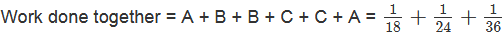Work done together = 2(A + B + C) = (4 + 3 + 2): 72 = 9: 72 = 1: 8

Work done together = A + B + C = 1: 16

Thus,

Together they can finish work in 16 days.

Question: 8

A and B can do a piece of work in 12 days; B and C can do it in 15 days; C and A can do it in 20 days.

How much time will A alone take to finish the work?

Solution:

It is given that:

Time taken by A + B to so the work = 12 days

Time taken by B + C to do the work = 15 days

Time taken by C + A = 20 days

Now,

Work done by A + B = 1: 12

Work done by B + C = 1: 15

Work done by C + A = 1: 20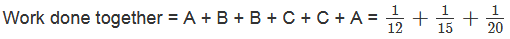Work done together = 2(A + B + C) = (5 + 4 + 3): 60 = 12: 60 = 1: 5

Work done together = A + B + C = 1: 10

Thus,

Work done by A alone = Work done by A + B + C – Work done by B + C

= 1: 10 – 1: 15

= (3 – 2): 30 = 1: 30

Hence, A alone can do the work in 30 days.

Question: 9

A, B and C can reap a field in 15(3/4) days; B, C and D in 14 days; C, D and A in 18 days; D, A and B in 21 days. In what time can A, b, C and D together reap it?

Solution:

It is given that:

Time taken by A + B + C to do the work = 15(3/4) days = 63/4 days.

Time taken by B + C + D to do the work = 14 days

Time taken by C + D + A to do the work = 18 days

Time taken by D + A + B to do the work = 21 days

Now,

Work done by A + B + C = 4: 63

Work done by B + C + D = 1: 14

Work done by C + D + A = 1: 18

Work done by D + A + B = 1: 21

Work done together = A + B + C + B + C + D + C + D + A + D + A + B =Work done together = 3(A + B + C + D) = (8 + 9 + 7 + 6): 126 = 30: 126 = 5: 21

Work done together = A + B + C + D = 5: 63

Thus,

Together they can do the work in 63: 5 days = 12(3/5) days

Question: 10

A and B can polish the floors of a building in 10 days. A alone can do 1/4 th of it in 12 days. In how many days can B alone polish the floor?

Solution:

It is given that:

A and B can polish the floors of the building in 10 days

Work done by A + B in 1 day = 1: 10

Now,

A alone can do 1/4 th of the work in 12 days.

Time taken by A alone to do the complete work = 4 × 12 = 48 days

So, work done by A in 1 day = 1: 48

Now,

Work done by B in 1 day = Work done by A + B in 1 day – Work done by A in 1 day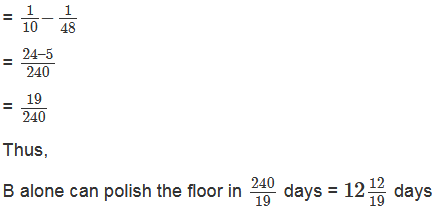Question: 11

A and B can finish a work in 20 days. A alone can do 1/5th of the work in 12 days. In how many days can B alone do it?

Solution:

It is given that:

A and B can finish the work in 20 days.

Work done by A + B in 1 day = 1: 20

Now,

A alone can do 1/5 th of the work in 12 days.

Time taken by A alone to do the complete work = 5 × 12 = 60 days.

So, work done by A in 1 day = 1: 60

Now,

Work done by B in 1 day = Work done by A + B in 1 day – Work done by A in 1 dayThus,

B alone can polish the floor in 30 days.

Question: 12

A and B can do a piece of work in 20 days and B in 15 days. They work together for 2 days and then A goes away. In how many days will B finish the remaining work?

Solution:

It is given that:

A finish the work in 20 days and B can finish the same work in 15 days

So,

Work done by A = 1: 20

Work done by B = 1: 15Question: 13

A can do a piece of work in 40 days and B in 45 days. They work together for 10 days and then B goes away. In how many days will B finish the remaining work?

Solution:

It is given that:

A can finish the work in 40 days and B can finish a work in 45 days.

So,

Work done by A in 1 day = 1: 40

Work done by B in 1 day = 1: 45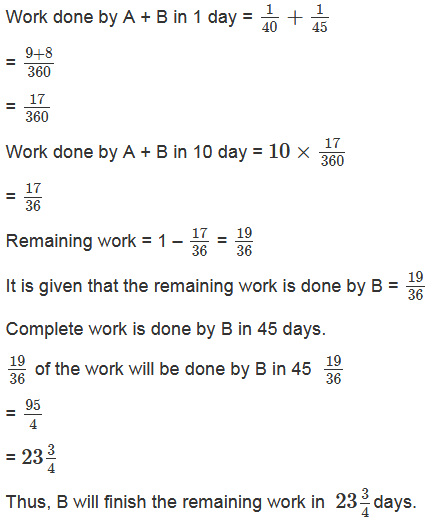Question: 14

Aasheesh can paint his doll in 20 minutes and his sister Chinki can do so in 25 minutes. They paint the doll together for 5 minutes. At this juncture they have a quarrel and Chinki withdrawn from painting. In how many minutes will Aasheesh finish the painting of the remaining doll?

Solution:

It is given that:

Aasheesh can paint a doll in 20 minutes, and Chinki can paint the same in 25 minutes.

Work done by Aasheesh in 1 day = 1: 20

Work done by Chinki in 1 day = 1: 25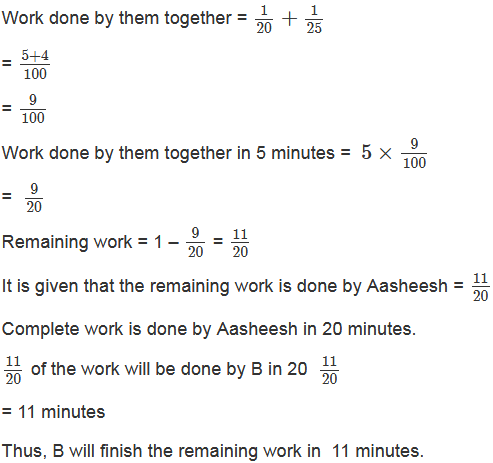Question: 15

A and B can do a piece of work in 6 days and 4 days, respectively. A started the work; worked at it for 2 days and then was joined by B. Find the total time taken to complete the work.

Solution:

It is given that:

A can do a work in 6 days, and B can do the same in 4 days.

Work done by A in 1 days = 1/6

Work done by B in 1 days = 1/4

Work done by A in 2 days = 2/6 = 1/3Question: 16

6 men can complete the electric fitting in a building in 7 days. How many days will be taken if 21 men do the job?

Solution:

It is given that:

6 men can complete a job in 7 days

Time taken by 1 man to complete the same job = 6 × 7 = 42 days.

Time taken by 21 men to complete the same job = 42/21 = 2 days

Question: 17

8 men can do a piece of work in 9 days. In how many days will 6 men do it?

Solution:

It is given that:

8 men can complete a job in 9 days.

Time taken by 1 men to complete the same job = 9 × 8 = 72 days.

Time taken by 6 men to complete the same job = 72/6 = 12 days.

Question: 18

Reema weaves 35 baskets in 25 days. In how many days will she weave 55 baskets?

Solution:

It is given that:

Reema weaves 35 baskets in 25 days.

Time taken by her to weave 1 basket = 25/35Question: 19

Neha types 75 pages in 14 hours. How many pages will she type in 20 hours?

Solution:

It is given that:

Neha types 75 pages in 14 hours

Number of pages typed by her in 1 hour = 14/75Question: 20

If 12 boys earn Rs. 840 in 7 days, what will 15 boys earn in 6 days?

Solution:

Earnings of 12 boys in 7 days = Rs. 840

Earnings of 12 boys in 1 day = Rs. 840/7 =  Rs. 120

Earnings of 1 boy in 1 day = Rs. 120/12 = Rs. 10

Earnings of 1 boy in 6 days = Rs. 10 × 6 = Rs. 60

Earnings of 15 boys in 6 days = Rs. 60 × 15 = Rs. 900

Question: 21

If 25 men earn Rs. 1000 in 10 days, how much will 15 men earn in 15 days?

Solution:

It is given that:

Earning of 25 men in 10 days = Rs. 1000

Earnings of 25 men in 1 day = Rs. 1000/10 = Rs. 100

Earnings of 1 men in 1 day = Rs. 100/25 = Rs. 4

Earnings of 1 men in 15 days = Rs. 4 × 15 = Rs. 60

Earnings of 15 men in 15 days = Rs. 60 × 15 = Rs. 900

Question: 22

Working 8 hours a day, Ashu can copy a book in 18 days. How many hours a day should he work so as to finish the work in 12 days?

Solution:

It is given that:

If Ashu works for 8 hours daily, he can finish the work in 18 days.

If he works for 1 hour daily, he can finish the work in 18 × 8 days or, 144 days.

Number of hours he should work daily to finish the work in 12 days = 144/12 = 12 hours.

Question: 23

If 9 girls can prepare 135 garlands in 3 hours, how much girls are needed to prepare 270 garlands in 1 hour?

Solution:

It is given that:

9 girls can prepare 135 garlands in 3 hours.

Number of garlands made by 9 girls in 1 hour = 135/3 = 45

Number of garlands made by 1 girls in 1 hours = 45/9 = 5

Number of girls required to make 270 garlands in 1 hour = 270/5 = 54 girls

Question: 24

A cistern can be filled by one tap in 8 hours, and by another in 4 hours. How long will it take to fill the cistern if both taps are opened together?

Solution:

It is given that:-

Time taken by the first tap to fill the cistern = 8 hours.

Time taken by the second tap to fill the cistern = 4 hours.

Work done by the first tap in 1 hour =1/8

Work done by second tap in 1 hour = ¼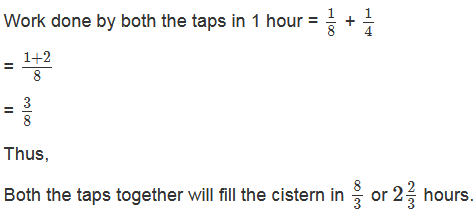Question: 25

Two taps A and B can fill an overhead tank in 10 hours and 15 hours, respectively. Both the taps are opened for 4 hours and then B is turned off. How much time will A take to fill the remaining tank?

Solution:

Pipe A can fill the tank in 10 hours, and pipe B can fill the tank in 15 hours.

In 1 hour, A can fill (1/10)th part of the tank.

In 1 hour, B can fill (1/15)th part of the tank.Question: 26

A pipe can fill a cistern in 10 hours. Due to a leak in the bottom it is filled in 12 hours. When the cistern is full, in how much time will it be emptied by the tank?

Solution:

It is given that:

When there is no leakage, the pipe can fill the cistern in 10 hours.

Thus, the pipe can fill (1/10)th part of the cistern in 1 hour.

When there is leakage, the pipe can fill the cistern in 12 hours.

Therefore, in case of leakage, the pipe can fill (1/12)th part of the cistern in 1 hour.

Thus, in one hour, due to leakage, (1/10) – (1/12) or (1/60)th part of the cistern is emptied.

Hence, the cistern will be emptied by the leakage in 60 hours.

Question: 27

A cistern has two inlets A and B which can fill it in 12 hours and 15 hours, respectively. An outlet can empty the full cistern in 10 hours. If all the three pipes are opened together in the empty cistern, how much time will they take to fill the cistern completely?

Solution:

It is given that:

Time taken by a tap A to fill the cistern = 12 hours.

Time taken by tap B to fill the cistern = 15 hours.

Let, C be the outlet that can empty the cistern in 10 hours.

Time taken by tap C to empty the cistern = 10 hours.

Now,Hence, the cistern will be filled completely in 20 hours if all the three taps are opened together.

Question: 28

A cistern can be filled by a tap in 4 hours and emptied by an outlet pipe in 6 hours. How long will it take to fill the cistern if both the tap and the pipe are opened together?

Solution:

It is given that:

Time taken by the tap to fill the cistern = 4 hours

Tap fills (1/4)th part of the cistern in 1 hour.

Time taken by the pipe to empty the cistern = 6 hours

Pipe empties out (1/6)th part of the cistern in 1 hour.

Thus,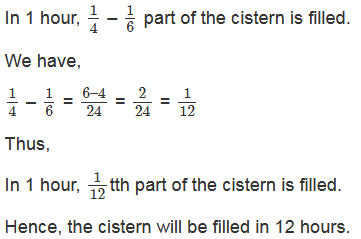### Course Features

• 728 Video Lectures
• Revision Notes
• Previous Year Papers
• Mind Map
• Study Planner
• NCERT Solutions
• Discussion Forum
• Test paper with Video Solution